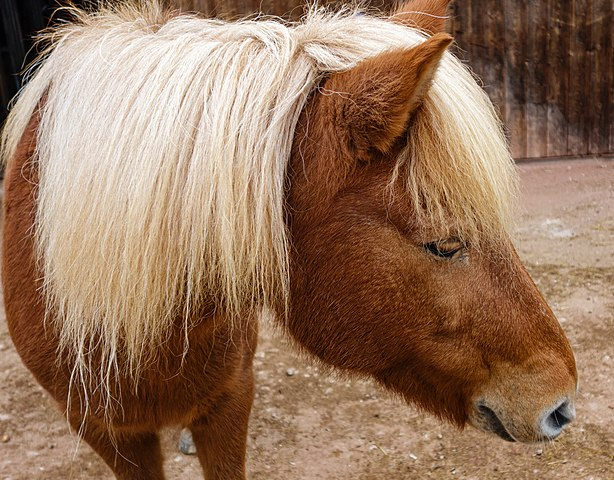# Horse Genetics

Students in AP Biology are given more challenging genetics problems than my freshman students. Crosses which involve two traits (AaBb x AaBb) are emphasized and students are taught how to use the mathematical method of solving the problems rather than the time-consuming Punnet squares.

I use this practice as a follow-up to the basic practice worksheet which covers both monohybrid and dihybrid crosses.

In this activity, students are given a short text describing traits that are thought to be genetic in origin: flaxen manes and type of gait. Flaxen manes and a pacing gait is thought to be recessive, though this may be an ideal time to discuss that other factors can play a role in the external phenotype of an organism.

Each problem asks students to determine the phenotypic ratio using a mathematical solution.

For example, if the cross is Ttff x ttFf, then the number of pacer, flaxen maned horses would be solved by multiplying. 1/2 pacer x 1/2 flaxen equals 1/4. 1/4 offspring in this cross will be pacers and have flaxen manes.Flaxen is a genetic trait in which the mane and tail of chestnut-colored horses are noticeably lighter than the body coat color, often a golden blonde shade. Manes and tails can also be a mixture of darker and lighter hairs.

HS-LS3-3 Apply concepts of statistics and probability to explain the variation and distribution of expressed traits in a population.

Posted

in

by